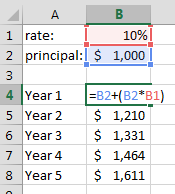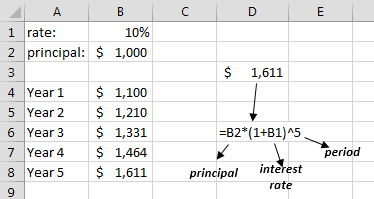# Compound Interest Calculation In Excel

There is not a preset function for compound interest calculation in Excel. But here is an easy formula which can easily substitute for that.

Compound interest is the term for a situation where interest of a principal is added to principal at the end of every period and new principal value becomes “actual principal + interest”. This means interest getting interest every period.

For example, if you deposit 1,000\$ to an account with 10% annual interest rate, you will get 100\$ interest at the end of the first year and your money will become 1,100\$. At the end of second year, your money will become 1,210\$ because principlal for 2nd year is 1,100\$. This will go on and your money will gain interest from principal + interests of previous periods (1000\$->1100\$-1210\$->1331\$->1464\$).

Since there isn’t a function preseent for compound interest calculation in Excel, you need to either calculate it period by period until you reach your desirred period like shown below. Which will be troublesome if you are calculation for 100th period.Or you can use the formula below for compound interest calculation in Excel with one step.

Total=principal*(1+interest_rate)^period

Lets calculate how much our 1,000\$ will become after 5 years with 10% annual interest rate in compound interest:As you can see, we can easily achieve the result that took 5 calculations before with only one formula.

#### You may also like...

This site uses Akismet to reduce spam. Learn how your comment data is processed.